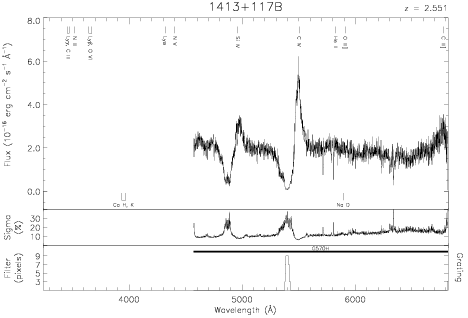Download dataset
TELESCOP= 'HST     '
INSTRUME= 'FOS     '
EQUINOX =               2000.0
DATE-OBS= '1993-06-27T09:56:27'
RA_PNT  =       213.9431281250
DEC_PNT =        11.4954888889
PA_PNT  =       218.4218000000
OBJECT  = '1413+117B'
ROOTNM01= 'Y1FB0303T'
GRNDMD01= 'SPECTROSCOPY'
DETECT01= 'AMBER   '
APERID01= 'B-1     '
FGWAID01= 'H57     '
ROOTNM02= 'Y1FB0304T'
GRNDMD02= 'SPECTROSCOPY'
DETECT02= 'AMBER   '
APERID02= 'B-1     '
FGWAID02= 'H57     '